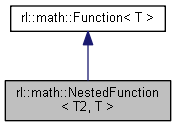Robotics Library  0.7.0
rl::math::NestedFunction< T2, T > Class Template Reference

#include <NestedFunction.h>

Inheritance diagram for rl::math::NestedFunction< T2, T >:[legend]

## Public Member Functions

NestedFunction (const Function< T2 > &inner, const Function< T > &outer)

virtual ~NestedFunction ()

NestedFunctionclone () const

operator() (const Real &x, const ::std::size_t &derivative=0) const
Evaluates the function or a derivative thereof for a given value x. More...Public Member Functions inherited from rl::math::Function< T >
Function ()

virtual ~Function ()

Real duration () const

Reallower ()

const Reallower () const

Realupper ()

const Realupper () const

## Private Attributes

const Function< T2 > & inner

const Function< T > & outerProtected Attributes inherited from rl::math::Function< T >
Real x0

Real x1

## ◆ NestedFunction()

template<typename T2 , typename T >
 rl::math::NestedFunction< T2, T >::NestedFunction ( const Function< T2 > & inner, const Function< T > & outer )
inline

## ◆ ~NestedFunction()

template<typename T2 , typename T >
 virtual rl::math::NestedFunction< T2, T >::~NestedFunction ( )
inlinevirtual

## ◆ clone()

template<typename T2 , typename T >
 NestedFunction* rl::math::NestedFunction< T2, T >::clone ( ) const
inlinevirtual

## ◆ operator()()

template<typename T2 , typename T >
 T rl::math::NestedFunction< T2, T >::operator() ( const Real & x, const ::std::size_t & derivative = 0 ) const
inlinevirtual

Evaluates the function or a derivative thereof for a given value x.

Some functions are only defined in the interval [lower(), upper()], and fail to evaluate outside of [lower() - FUNCTION_BOUNDARY, upper() + FUNCTION_BOUNDARY]. In Debug mode, this is signaled by failing asserts. In Release mode, the function is evaluated if algebraically possible, or will return an empty ArrayX otherwise. Some functions are not indefinitely often differentiable, and will return a NaN array for all higher orders.

Parameters
 [in] x Input value of the function or derivative [in] derivative Order of the derivative to be evaluated

Implements rl::math::Function< T >.

## ◆ inner

template<typename T2 , typename T >
 const Function& rl::math::NestedFunction< T2, T >::inner
private

## ◆ outer

template<typename T2 , typename T >
 const Function& rl::math::NestedFunction< T2, T >::outer
private

The documentation for this class was generated from the following file: